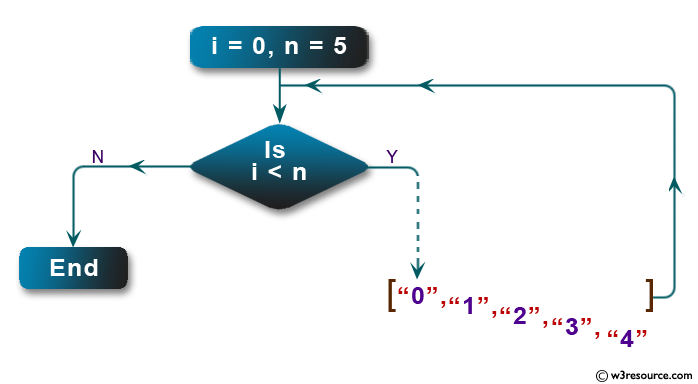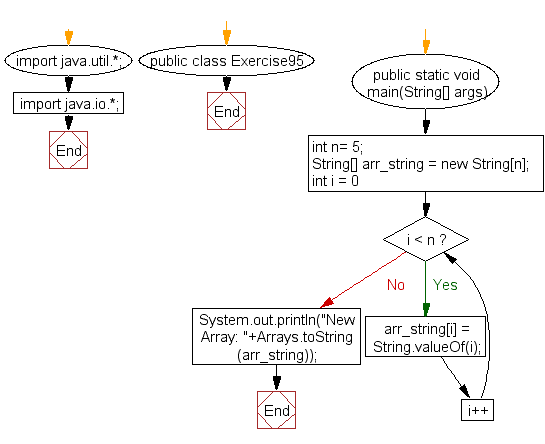﻿ Java exercises: Create an array of string values - w3resource# Java Exercises: Create an array of string values

## Java Basic: Exercise-95 with Solution

Write a Java program to create an array (length # 0) of string values. The elements will contain "0", "1", "2" … through ... n-1.

Pictorial Presentation:Sample Solution:

Java Code:

``````import java.util.*;
import java.io.*;
public class Exercise95 {
public static void main(String[] args)
{
int n= 5;
String[] arr_string = new String[n];

for(int i = 0; i < n; i++)
arr_string[i] = String.valueOf(i);
System.out.println("New Array: "+Arrays.toString(arr_string));
}
}
```
```

Sample Output:

```New Array: [0, 1, 2, 3, 4]
```

Flowchart:Java Code Editor:

What is the difficulty level of this exercise?

Test your Programming skills with w3resource's quiz.

﻿

## Java: Tips of the Day

getEnumMap

Converts to enum to Map where key is the name and value is Enum itself.

```public static <E extends Enum<E>> Map<String, E> getEnumMap(final Class<E> enumClass) {
return Arrays.stream(enumClass.getEnumConstants())
.collect(Collectors.toMap(Enum::name, Function.identity()));
}
```

Ref: https://bit.ly/3xXcFZt